# 高速切削镍基高温合金条件下影响毛刺因素的研究Study on the Influence Factors of Burr in High Speed Cutting of Nickel-Base Superalloy

DOI: 10.12677/MET.2020.92009, PDF, HTML, XML, 下载: 124  浏览: 197  国家自然科学基金支持

Abstract: Nickel-base superalloy is a kind of hard to machine metal material. It is difficult to guarantee the quality of workpiece after using this material. The quality of workpiece is an important content of high-speed machining, which has a great influence on the wear resistance, corrosion resistance and heat conduction performance of machined parts. The factors that affect the quality of the workpiece in high speed cutting of high temperature nickel-base alloy are studied by the method of cutting experiment and simulation analysis. Based on the analysis of the metallographic picture of the cutting root obtained from the cutting experiment and the experimental phenomenon, it is concluded that the larger the cutting depth is, the higher the burr height is. In the case of a certain cutting depth, the higher the cutting speed, the more difficult to form retained burr, the better the quality of the workpiece.

1. 引言

2. 建模与实验

2.1. 多晶体有限元模型的建立Figure 1. Polycrystalline model

2.2. 材料的本构方程

${\stackrel{¯}{\sigma }}_{e}=\left[A+B{\left({\stackrel{¯}{\epsilon }}_{e}\right)}^{n}\right]\left[1+C\mathrm{ln}\left(\frac{{\stackrel{˙}{\stackrel{¯}{\epsilon }}}_{e}}{{\stackrel{˙}{\stackrel{¯}{\epsilon }}}_{0}}\right)\right]\left[1-{\left(\frac{T-{T}_{0}}{{T}_{melt}-{T}_{0}}\right)}^{m}\right]$ (1)

Inconel 718合金的Johnson-Cook材料本构模型的参数如表1所示Table 1. Parameters of Johnson cook model for Inconel 718 Alloy

2.3. 实验过程Table 2. Main chemical components of Inconel 718 (wt%)Table 3. Physical and mechanical properties of Inconel 718 (20˚C)

3. 结果与讨论

3.1. 切削过程中晶粒的变化

${\tau }_{r}=\tau \sqrt{\frac{L}{r}}$ (2)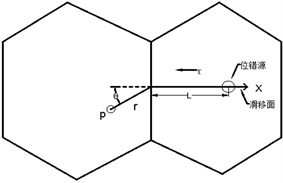Figure 2. Dislocation slip model of adjacent crystal

${\tau }_{r}=\tau {\left(\frac{d}{r}\right)}^{\frac{1}{2}}$ (3)

${\tau }_{r}$ 随着晶粒直径d与塞积头与相邻晶粒内最近的位错源之间的距离r的变化情况如图3所示：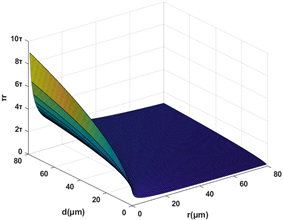Figure 3. The change of τr with r and d

${\tau }_{r}=\left(\tau -{\tau }^{\prime }\right){\left(\frac{d}{r}\right)}^{\frac{1}{2}}$ (4)

${\tau }_{r}$ 达到能触发相邻晶粒的滑移(即位错源开动)所需的最小切应力 ${\tau }_{c}$ 时，变形就可继续，此时的外加切应力 $\tau$，就是多晶体的屈服应力 ${\tau }_{s}$，即 ：

${\tau }_{c}=\left({\tau }_{s}-{\tau }^{\prime }\right){\left(\frac{d}{r}\right)}^{\frac{1}{2}}$ (5)

${\tau }_{s}={\tau }^{\prime }+{\tau }_{c}{\left(\frac{d}{r}\right)}^{\frac{1}{2}}={\tau }^{\prime }+{k}^{\prime }{d}^{-\frac{1}{2}}$ (6)

${\sigma }_{s}={\sigma }^{\prime }+{k}^{\prime }{d}^{-\frac{1}{2}}$ (7)

3.2. 毛刺产生机理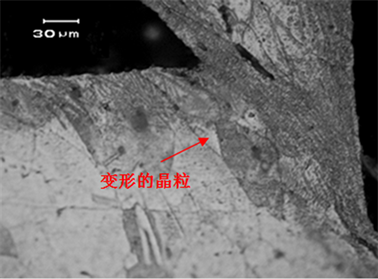Figure 4. Metallographic picture of cutting root of deformed grain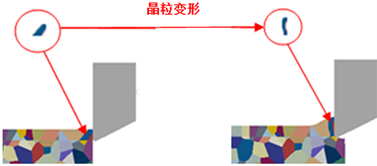Figure 5. Grain deformation in cutting simulation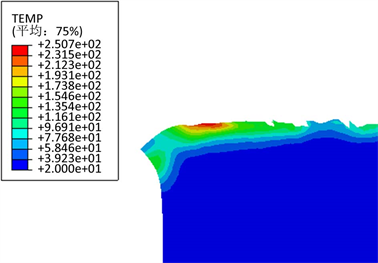Figure 6. Increase of grain temperature in shear zone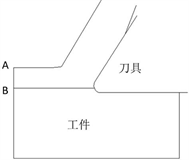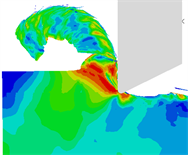(a)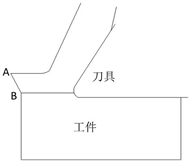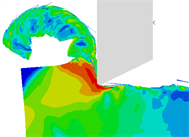(b)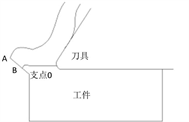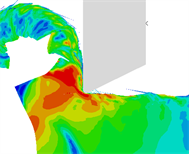(c)

Figure 7. Three stages of burr formation: (a) The first stage; (b) The second stage; (c) The third stageFigure 8. Fourth shear zone

3.3. 切削厚度对毛刺高度的影响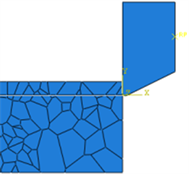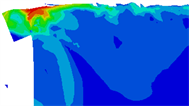(a)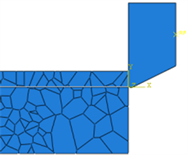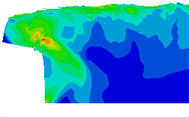(b)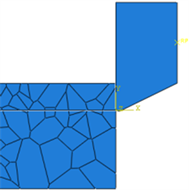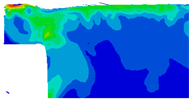(c)

Figure 9. Simulation phenomenon of different cutting thickness: (a) Cutting thickness is 0.25; (b) Cutting thickness is 0.5; (c) Cutting thickness is 0.75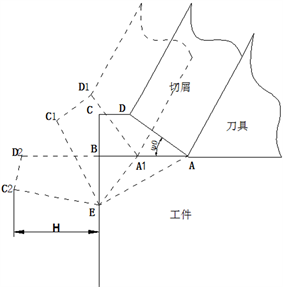Figure 10. Process of burr formation

$\phi =\frac{\pi }{4}-\left(\beta -{\gamma }_{0}\right)$ (8)

$a=\text{BE}=s\mathrm{tan}{\phi }_{0}$ (9)

$b=\text{CD}=s-{a}_{p}\mathrm{tan}\phi$ (10)

$H=\frac{b+s}{a+b}{a}_{p}$ (11)

$H=\frac{2s-{a}_{p}\mathrm{tan}{\phi }_{0}}{s\mathrm{tan}{\phi }_{0}+s-{a}_{p}\mathrm{tan}\phi }{a}_{p}$ (12)

$\frac{\partial H}{\partial {a}_{p}}>0$ (13)

3.4. 切削速度对毛刺形态的影响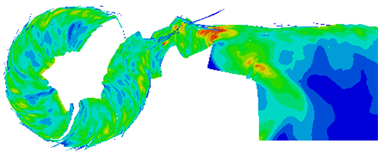(a)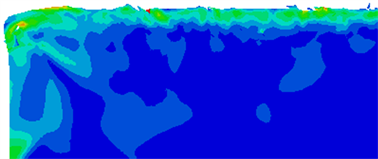(b)

Figure 11. Simulation results at different speeds: (a) At 120 m/min; (b) At 200 m/min

4. 总结

1) 所建立的高温镍基合金多晶体有限元模型能够很好地反映出金属材料的内部情况，使其更加接近真实的金属材料微观形貌。

2) 通过对不同切削深度条件下产生的毛刺进行分析，发现切削深度越大，形成的毛刺高度越高。这是由于切削深度增加，会使切屑的变形以及增大，最终刀具切出工件时，生成的毛刺体积也随之增大，从而使毛刺的高度增加。

3) 通过对不同切削速度条件下产生的毛刺进行分析，发现切削速度越大，越不容易形成滞留毛刺。这是由于切削速度的增加，会使切削力也随之增大。当切削速度较小时，切削力不足以使产生的切屑与工件基体脱离。当切削速度增加以后，切削力增大，工件末端变形增大，第四变形区的应力值也随之增加，工件末端发生断裂，切屑脱离工件基体，形成毛刺。所以切削速度越大，越不容易形成滞留毛刺。

  陈世平, 罗辑, 石军. 金属切削加工中的毛刺问题[J]. 机械设计与制造, 2004(1): 99-100.  Hambli, R. (2002) Prediction of Burr Height Formation in Blanking Processes Using Neural Network. International Journal of Mechanical Sciences, 44, 2089-2102.  Martellotti, M. (1941) An Analysis of the Milling Process.  Lee, K.Y., Kang, M.C., Jeong, Y.H., et al. (2001) Simulation of Surface Roughness and Profile in High-Speed End Milling. Journal of Materials Processing Technology, 113, 410-415. https://doi.org/10.1016/S0924-0136(01)00697-5  Omar, O.E.E.K., El-Wardany, T., Ng, E., et al. (2007) An Improved Cutting Force and Surface Topography Prediction Model in End Milling. International Journal of Machine Tools & Manufacture, 47, 1263-1275. https://doi.org/10.1016/j.ijmachtools.2006.08.021  Arizmendi, M., Campa, F.J., Fern, N.J., et al. (2009) Model for Surface Topography Prediction in Peripheral Milling Considering Tool Vibration. CIRP Annals Manufacturing Technology, 58, 93-96. https://doi.org/10.1016/j.cirp.2009.03.084  魏立峰. 光学零件超精密铣削加工表面形貌预测与仿真[D]: [硕士学位论文]. 武汉: 华中科技大学, 2008.  李成锋. 介观尺度铣削力与表面形貌建模及工艺优化研究[D]: [博士学位论文]. 上海: 上海交通大学, 2008.  Luo, X., Cheng, K. and Ward, R. (2005) The Effects of Machining Process Variables and Tooling Characterisation on the Surface Generation. The International Journal of Advanced Manufacturing Technology, 25, 1089-1097. https://doi.org/10.1007/s00170-003-1943-0  李荣彬, 张志辉, 李建广. 超精密加工的三维表面形貌预测[J]. 中国机械工程, 2000, 11(8): 845-848.  范依航, 王涛, 郝兆朋, 等. 基于多尺度仿真的Inconel 718切削过程研究[J]. 制造技术与机床, 2017(9): 114-117.  Zhu, Z.C. (2014) Martensitic (20). Shanghai University of Science, Shanghai.  Hall, E.O. (1970) Yield Point Phenomena in Metals and Alloys. Plenum Press, New York. https://doi.org/10.1007/978-1-4684-1860-6